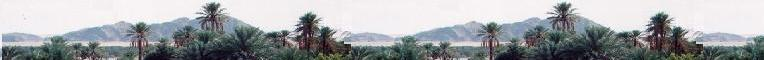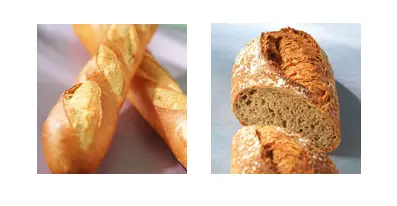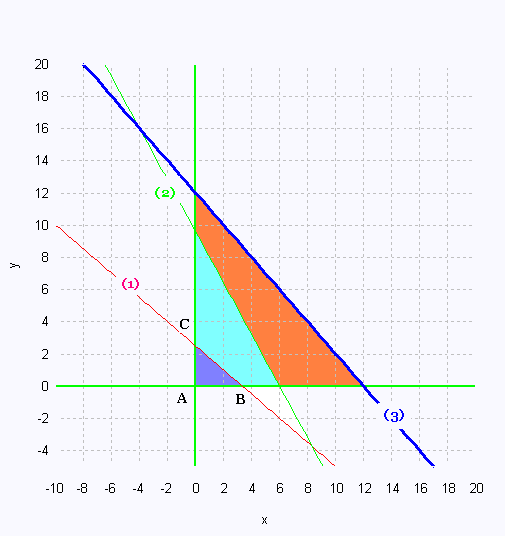# Mathematics 2: Linear optimization ExamplesA baker makes two kinds of bread of high quality: wholewheat bread and French bread. One wholemeal bread costs 3$and one French bread costs 2$.

For 40 wholewheat breads, It takes for an hour and a half to be prepared, 40 minutes to be baked, and 10 minutes to be packed and sold.

For 50 French breads, It takes for them two hours to be prepared, 25 minutes to be baked, and 10 minutes to be packed and sold.

The time is limited to 5 hours to prepare these breads, 4 hours to bake them and 2 hours to pack and sell them.

The backer wants to work less and earn more making a maximum profit. To do so, how many breads of each kind should he produce?

step 1 :
x : 1 set of 40 wholewheat breads
y : 1 set of 50 French breads

x ≥ 0
y  ≥ 0
(1) 90 x + 120 y ≤ 5 x 60 = 300
(2) 40 x + 25 y ≤ 4 x 60 = 240
(3) 10 x + 10 y ≤ 2 x 60 = 120

Step 2:
We translate the five constraints in a Cartesian
plane.
Using the above inequality, we identify the polygon
of constraints that will contain all the areas of each
constraints.

Isolate the variables y in the three equations (1),
(2), and (3):

(1)  y ≤ - 3/4 x + 5/2
(2)  y ≤  - 8/5 x + 48/5
(3)  y ≤  - x + 12

Plot the graph:The vertices of the polygon of constraints
determine the minimum or maximum value of the
function to be optimized.

From the polygon of constraints, we consider the
three vertices.

Vertex A: (0,0)
Vertex B: (0,5/2)
Vertex C: (10/3,0)

Step 4:
We write the equation that will use the found vertices
and thus answer the question of the problem.
We want the maximum profit:

The function objective Z is

Z =  3 x 40 x + 2 x 50 y = 120 x + 100 y

Step 5:
In a table, we use the function to optimize Z
and perform a calculation using each found vertex.

Vertex 			Z ($) (0, 0) 0 (0, 5/2) 250 (10/3, 0) 400 Step 6: We find one maximum value to maximize the profit. It is 400$.

Answer: To get the maximum benefit, The baker will use
10/3 sets of 40 = 133 wholewheat breads, and no FrenchWeb ScientificSentence

 chimie labs | scientific sentence | java | php | green cat | contact |